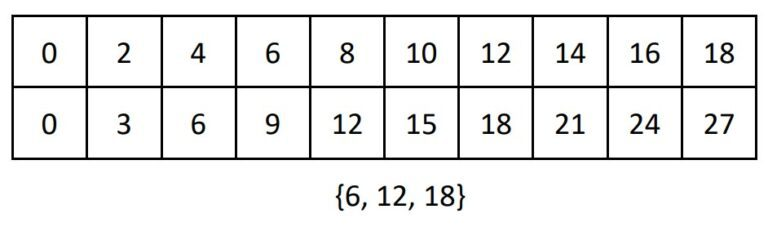# 4th Grade, Activity 87: Gain Familiarity with Factors and Multiples

Using whole numbers in the range 1–100 (Factors and Multiples).

c. Determine whether a given whole number is a multiple of a given one-digit number.

### Identify the Common Multiples

• Group: Whole Class/Pairs
• Materials: Identify the Common Multiples sheet

Invite two volunteers to the board. Instruct one student to write ten multiples of 2 and ask the other student to write ten multiples of 3 as shown in the example below.Ask the class if they can identify any of the numbers that are the same in both lists. Write the phrase common multiples on the board to describe the numbers that are the same in both lists. Explain that if two numbers have some multiples that are the same, the two numbers have common multiples. Provide more examples as needed.

Identify one student as Student A and identify the other student as Student B. Issue an Identify the Common Multiples sheet to each student. Instruct the student to find ten multiples of the number assigned to Student A and have the other student find ten multiples of the number assigned to Student B. Instruct both students to write each other’s multiples on their papers. Students then identify the multiples that are common to both numbers. When students complete the exercises, have them compare their answers with another pair.